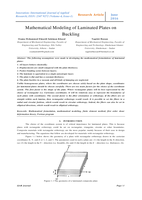اسم الكتاب

# Mathematical Modeling of Laminated Plates on Buckling

القسم

ركن الهندسة الميكانيكية - Mechanical Engineering

عن الكتاب

The following assumptions were made in developing the mathematical formulations of laminated plates: 1. All layers behave elastically; 2. Displacements are small compared with the plate thickness; 3. Perfect bonding exists between layers; 4. The laminate is equivalent to a single anisotropic layer; 5. The plate is flat and has a constant thickness; 6. The plate buckles in a vacuum and all kinds of damping are neglected. Unlike homogeneous plates, where the coordinates are chosen solely based on the plate shape, coordinates for laminated plates should be chosen carefully. There are two main factors for the choice of the coordinate system. The first factor is the shape of the plate. Where rectangular plates will be best represented by the choice of rectangular (i.e. Cartesian) coordinates. It will be relatively easy to represent the boundaries of such plates with coordinates. The second factor is the fiber orientation or orthotropy. If the fibers are set straight within each lamina, then rectangular orthotropy would result. It is possible to set the fibers in a radial and circular fashion, which would result in circular orthotropy. Indeed, the fibers can also be set in elliptical directions, which would result in elliptical orthotropy.

صاحب الكتاب

عدد القراء

1829

عدد مرات التحميل

22

التقييم

تاريخ الأضافة

2018-05-2811:09 AM

نوع الملف

application / pdf

حجم الكتاب

0.68 ميجا بايت

التعليقات

{{ comment.created_at }}

{{ whoCommented(comment) }}

{{ comment.comment }}